## MAGP (Example results)

Let X be a Gaussian process with mean and covariance functions denoted by: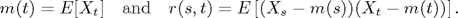We assume regularity conditions imposed in Mercadier (2006).

Let M(a,b) denotes the maximum of X over the interval [a,b]: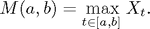MAGP computes (by lower and upper bounds) the distribution of the maximum M(a,b) when X is centered.
The estimation of P[M(a,b)>u] is given by the command magp(r,a,b,u,option). Consequently, this toolbox allows for instance:

• the approximation of the pdf of M(a,b);
• the analysis of the behaviour of {M(0,T)>u} when T varies (see below);
• ...

Example 1 -- Stationary case with: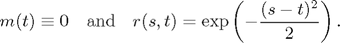```syms x y
r=exp(-(x-y)^2/2);

% Bounds for P( M(0,1)> 1 ):

magp(r,0,1,1,500)
ans =
0.2533    0.2541

% Plot the bounds of the function T -> P( M(0,T) > 1 ) obtained by MAGP: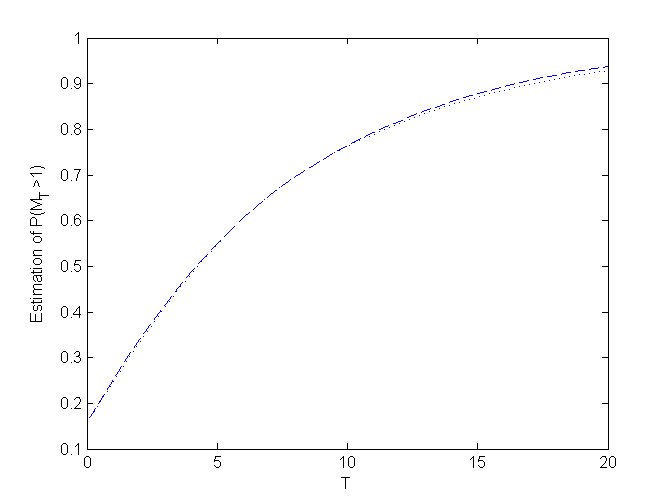```

Example 2 -- Non stationary case with: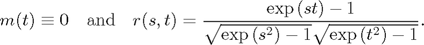```syms x y
r=(exp(x*y)-1)/sqrt((exp(x^2)-1)*(exp(y^2)-1));

% Bounds for P( M(0,1) > 1 ):

magp(r,0,1,1,500)
ans =
0.2299    0.2297

% Plot the bounds of the function T -> P( M(0,T) > 1 ) obtained by MAGP: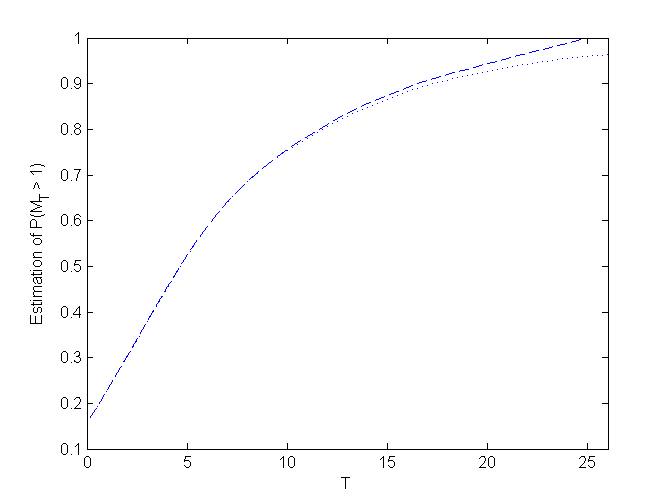```